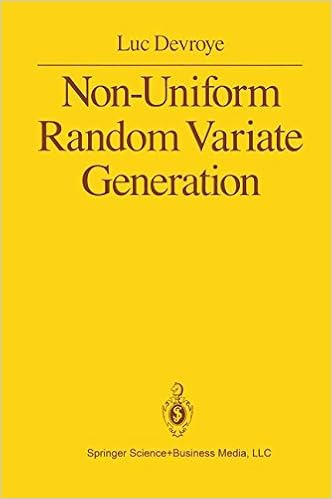## ratio-of-uniforms [#2]Following my earlier post on Kinderman’s and Monahan’s (1977) ratio-of-uniform method, I must confess I remain quite puzzled by the approach. Or rather by its consequences. When looking at the set A of (u,v)’s in R⁺×X such that 0≤u²≤ƒ(v/u), as discussed in the previous post, it can be represented by its parameterised boundary

u(x)=√ƒ(x),v(x)=x√ƒ(x)    x in X

Similarly, since the simulation from ƒ(v/u) can also be derived [check Luc Devroye’s Non-uniform random variate generation in the exercise section 7.3] from a uniform on the set B of (u,v)’s in R⁺×X such that 0≤u≤ƒ(v+u), on the set C of (u,v)’s in R⁺×X such that 0≤u³≤ƒ(v/√u)², or on the set D of (u,v)’s in R⁺×X such that 0≤u²≤ƒ(v/u), which is actually exactly the same as A [and presumably many other versions!, for which I would like to guess the generic rule of construction], there are many sets on which one can consider running simulations. And one to pick for optimality?! Here are the three sets for a mixture of two normal densities:For instance, assuming slice sampling is feasible on every one of those three sets, which one is the most efficient? While I have no clear answer to this question, I found on Sunday night that a generic family of transforms is indexed by a differentiable  monotone function h over the positive half-line, with the uniform distribution being taken over the set

H={(u,v);0≤u≤h(f(v/g(u))}

when the primitive G of g is the inverse of h, i.e., G(h(x))=x. [Here are the slides I gave at the Warwick reading group on Devroye’s book last week:]

### 3 Responses to “ratio-of-uniforms [#2]”

1. […] suggested in the exercise, generating order statistics is straightforward, since, as suggested by Devroye, see Section V.3.3, the i-th spacing is an Exponential variate with rate (N-i+1). This is so fast […]

2. […] article was first published on R – Xi'an's Og, and kindly contributed to […]

3. […] Please comment on the article here: R – Xi’an’s Og […]

This site uses Akismet to reduce spam. Learn how your comment data is processed.